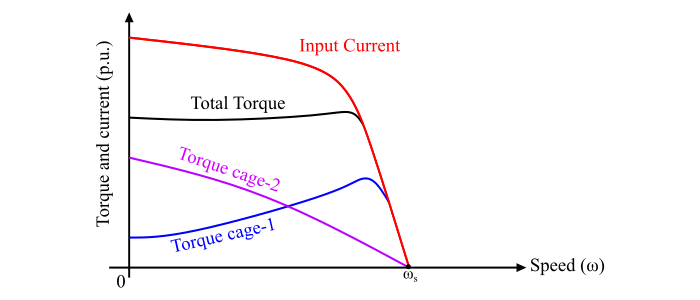# Torque-Slip Characteristics of Double-Cage Induction Motor and Comparison of Cage Torques

## Torque-Slip Characteristics of Double-Cage Induction Motor

In a double-cage induction motor, it is assumed that the two cages develop two separate torques. Thus, the total torque developed in the double-cage induction motor is equal to the sum of the two cage torques. The torque-slip characteristics of the two cages and the total torque of the motor is shown in the figure.By changing the individual cage resistances and leakage reactances, the resultant torque-speed characteristics can be modified according to the requirement. The resistances can be changed by changing the cross-sectional area of the rotor bars while the leakage reactance can be changed by changing the width of the slot openings and the depth of the inner cage.

## Comparison of Cage Torques of Double-Cage Induction Motor

Refer the equivalent circuit of the double-cage induction motor shown in Figure-1 −The per phase power developed by the inner rotor cage is given by,

$$\mathrm{𝑃_{𝑑𝑖} =\frac{𝐼′_{𝑟𝑖}^{2}𝑅'_{𝑟𝑖}}{𝑠}… (1)}$$

And the per phase power developed by the outer rotor cage is,

$$\mathrm{𝑃_{𝑑𝑜} =\frac{𝐼′_{𝑟𝑜}^2𝑅'_{𝑟𝑜}}{𝑠}… (2)}$$

Therefore, the total power developed per phase by both the cages is,

$$\mathrm{𝑃_𝑑 = 𝑃_{𝑑𝑖} + 𝑃_{𝑑𝑜} =\frac{𝐼′_{𝑟𝑖}^{2}𝑅'_{𝑟𝑖}}{𝑠}+\frac{𝐼′_{𝑟𝑜}^2𝑅'_{𝑟𝑜}}{𝑠}… (3)}$$

Also,

$$\mathrm{𝐼′_{𝑟𝑜} =\frac{𝐸′_𝑟}{𝑍′_{𝑟𝑜}}… (4)}$$

$$\mathrm{𝐼′_𝑟𝑖 =\frac{𝐸′_𝑟}{𝑍′_{𝑟𝑖}}… (5)}$$

$$\mathrm{𝑍′_{𝑟𝑜} = \sqrt{(\frac{𝑅′_{𝑟𝑜}}{𝑠})^2+ (𝑋′_{𝑟𝑜})^2} … (6)}$$

And,

$$\mathrm{𝑍′_{𝑟𝑖} = \sqrt{(\frac{𝑅′_{𝑟i}}{𝑠})^2+ (𝑋′_{𝑟i})^2} … (7)}$$

Now, let,

• τ𝑑𝑖 = Torque developed by the inner cage
• τ𝑑𝑜 = Torque developed by the outer cage
• τ𝑑 = Total torque developed by the two cages

Thus, the total power developed and hence the total developed torque is given by,

$$\mathrm{𝑃_𝑑 = 2𝜋𝑛_𝑠\tau_𝑑}$$

$$\mathrm{\therefore \tau_𝑑 =\frac{𝑃_𝑑}{2𝜋𝑛_𝑠}}$$

$$\mathrm{⇒ 𝜏𝑑 =\frac{1}{2𝜋𝑛_𝑠}(\frac{𝐼′_{𝑟𝑖}^2𝑅'_{𝑟𝑖}}{𝑠}+\frac{𝐼′_{𝑟𝑜}^2𝑅'_{𝑟𝑜}}{𝑠}) … (8)}$$

Hence, the ratio of the outer cage torque and the inner cage will be,

$$\mathrm{\frac{\tau_{𝑑𝑜}}{\tau_{𝑑𝑖}}=\frac{(\frac{𝑅′_{𝑟𝑜}}{𝑠})^2+ (𝑋′_𝑟𝑜)^2}{(\frac{𝑅′_{𝑟o}}{𝑠})^2+ (𝑋′_{𝑟𝑖})^2}… (9)}$$